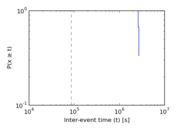This bipartite network contains a snapshot of the Google+ social structure. Left nodes represent users and right nodes represent attributes. An edge links a user to a attribute which he may share with other users.

 Code IA Internal name stevgong_attribute Name Google+ (IMC12) Data source http://www.cs.berkeley.edu/~stevgong/gplus.html Availability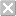Dataset is not available for download Consistency checkDataset passed all tests Category Feature network Dataset timestamp 2012 Node meaning User, attribute Edge meaning Attribute-link Network formatBipartite, undirected Edge typeUnweighted, no multiple edges Temporal dataEdges are annotated with timestamps

Statistics

 Size n = 10,442,421 Left size n1 = 5,998,790 Right size n2 = 4,443,631 Volume m = 20,592,962 Wedge count s = 31,413,709,466 Claw count z = 310,331,918,210,903 Cross count x = 4,592,753,920,185,512,448 Maximum degree dmax = 95,165 Maximum left degree d1max = 423 Maximum right degree d2max = 95,165 Average degree d = 3.944 10 Average left degree d1 = 3.432 85 Average right degree d2 = 4.634 26 Fill p = 7.725 33 × 10−7 Size of LCC N = 9,363,023 Diameter δ = 24 50-Percentile effective diameter δ0.5 = 5.482 73 90-Percentile effective diameter δ0.9 = 7.315 80 Median distance δM = 6 Mean distance δm = 6.046 39 Gini coefficient G = 0.579 175 Balanced inequality ratio P = 0.290 747 Left balanced inequality ratio P1 = 0.342 269 Right balanced inequality ratio P2 = 0.181 707 Relative edge distribution entropy Her = 0.893 300 Power law exponent γ = 2.492 22 Tail power law exponent γt = 1.961 00 Degree assortativity ρ = −0.017 463 1 Degree assortativity p-value pρ = 0.000 00 Spectral norm α = 309.460 Spectral separation |λ1[A] / λ2[A]| = 1.316 52 Controllability C = 4,592,127 Relative controllability Cr = 0.439 757

Plots

Degree distribution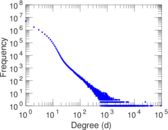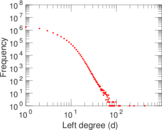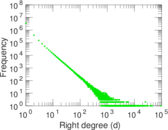Cumulative degree distribution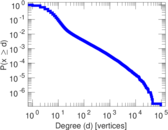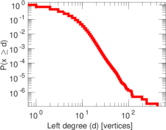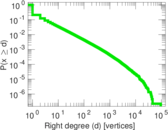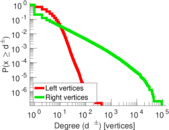Lorenz curve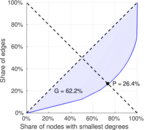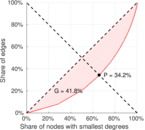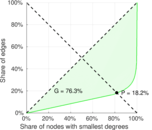Spectral distribution of the adjacency matrix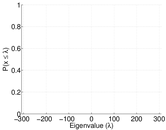Spectral distribution of the normalized adjacency matrix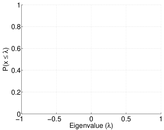Spectral distribution of the Laplacian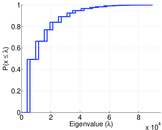Spectral graph drawing based on the adjacency matrix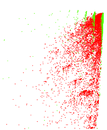Spectral graph drawing based on the normalized adjacency matrix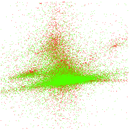Zipf plot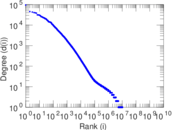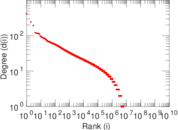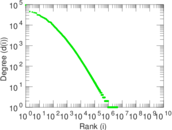Hop distribution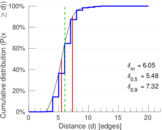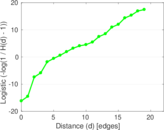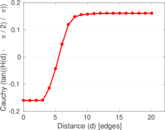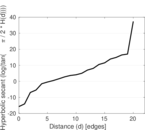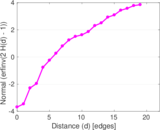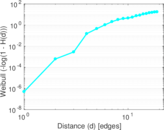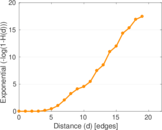Temporal distribution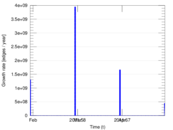Diameter/density evolution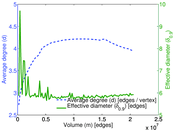Inter-event distribution